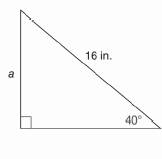Chapter 11.CR, Problem 1CRElementary Geometry For College St...

7th Edition
Alexander + 2 others
ISBN: 9781337614085

Solutions

Chapter
SectionElementary Geometry For College St...

7th Edition
Alexander + 2 others
ISBN: 9781337614085
Textbook Problem

In Review Exercises 1 to 4, state the ratio needed, and use it to find the measure of the indicated line segment to the nearest tenth of a unit.To determine

To find:

The measure of the indicated line segment to the nearest tenth of a unit.

Explanation

Procedure used:

In any right triangle ABC with mA=θ we have the following ratios.

sinθ=OppositeHypotenuse

Calculation:

Given:

Still sussing out bartleby?

Check out a sample textbook solution.

See a sample solution

The Solution to Your Study Problems

Bartleby provides explanations to thousands of textbook problems written by our experts, many with advanced degrees!

Get Started

Solve the equations in Exercises 126. (x3+1)x+1(x3+1)2x+1=0

Finite Mathematics and Applied Calculus (MindTap Course List)

In Exercises 2340, find the indicated limit. 33. limx22x+1x+2

Applied Calculus for the Managerial, Life, and Social Sciences: A Brief Approach

Compute C7,7.

Understanding Basic Statistics

Evaluate the integral. 68. x2x6+3x3+2dx

Single Variable Calculus: Early Transcendentals